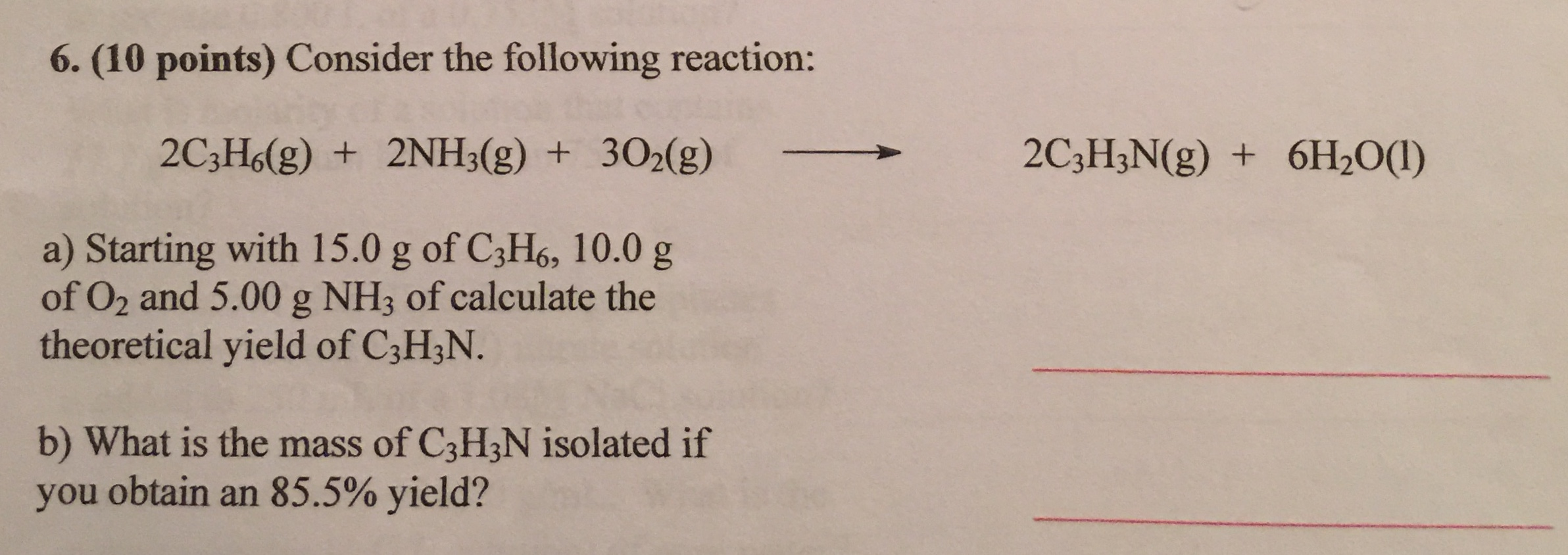# Consider the following reaction: 2C3H6 (g) + 2NH3 (g) + 3 O2 (g) 2C3H3N (g) + 6H2O (l) starting with 15.0g of C3H6, 10 g of O2 and 5.00 g NH3 of calculate the theoretical yield of C3H3N. What is the mass of C3H3N isolated if you obtain an 85.5% yield